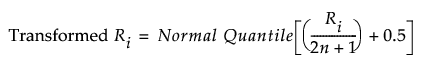Basic Analysis > Oneway Analysis > Analysis of Means Methods > Analysis of Means for Location
Publication date: 05/24/2021

## Analysis of Means for Location

You can test whether groups have a common mean or center value using the following options:

ANOM

ANOM with Transformed Ranks

### ANOM

Use ANOM to compare group means to the overall mean. This method assumes that your data are approximately normally distributed. See Example of an Analysis of Means Chart.

### ANOM with Transformed Ranks

This is the nonparametric version of the ANOM analysis. Use this method if your data is clearly non-normal and cannot be transformed to normality. ANOM with Transformed Ranks compares each group’s mean transformed rank to the overall mean transformed rank. The ANOM test involves applying the usual ANOM procedure and critical values to the transformed observations.

#### Transformed Ranks

Suppose that there are n observations. The transformed observations are computed as follows:

Rank all observations from smallest to largest, accounting for ties. For tied observations, assign each one the average of the block of ranks that they share.

Denote the ranks by R1, R2,..., Rn.

The transformed rank corresponding to the ith observations is:The ANOM procedure is applied to the values Transformed Ri. Since the ranks have a uniform distribution, the transformed ranks have a folded normal distribution. See Nelson et al. (2005).